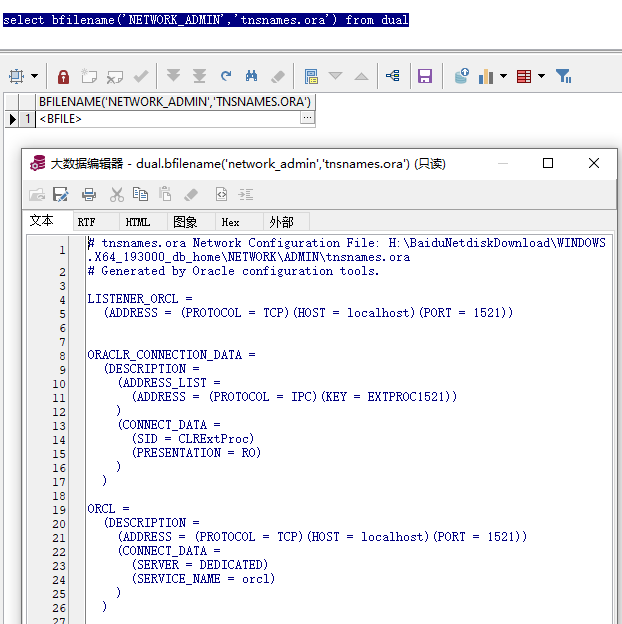# 19.approx_count_distinct

``````select col1,approx_count_distinct(col2) from tab1 group by col1;
``````

# 21.dbms_crypto

``````select sys.dbms_crypto.Hash(UTL_RAW.CAST_TO_RAW('123') ,2 ) from dual;
--或
select sys.dbms_crypto.Hash(UTL_I18N.STRING_TO_RAW ('123', 'AL32UTF8') ,2 ) from dual;
``````

hash函数的第一个参数可以传入raw、blob、clob类型，也就是说varchar2和number不能直接传入，传入的话可能会被解析成十六进制数据。既然支持blob，那么就当然能变向的支持二进制文件了。

-- Hash Functions
HASH_MD4 CONSTANT PLS_INTEGER := 1;
HASH_MD5 CONSTANT PLS_INTEGER := 2;
HASH_SH1 CONSTANT PLS_INTEGER := 3;
HASH_SH256 CONSTANT PLS_INTEGER := 4;
HASH_SH384 CONSTANT PLS_INTEGER := 5;
HASH_SH512 CONSTANT PLS_INTEGER := 6;

-- MAC Functions
HMAC_MD5 CONSTANT PLS_INTEGER := 1;
HMAC_SH1 CONSTANT PLS_INTEGER := 2;
HMAC_SH256 CONSTANT PLS_INTEGER := 3;
HMAC_SH384 CONSTANT PLS_INTEGER := 4;
HMAC_SH512 CONSTANT PLS_INTEGER := 5;
-- Block Cipher Algorithms
ENCRYPT_DES CONSTANT PLS_INTEGER := 1; -- 0x0001
ENCRYPT_3DES_2KEY CONSTANT PLS_INTEGER := 2; -- 0x0002
ENCRYPT_3DES CONSTANT PLS_INTEGER := 3; -- 0x0003
ENCRYPT_AES CONSTANT PLS_INTEGER := 4; -- 0x0004
ENCRYPT_PBE_MD5DES CONSTANT PLS_INTEGER := 5; -- 0x0005
ENCRYPT_AES128 CONSTANT PLS_INTEGER := 6; -- 0x0006
ENCRYPT_AES192 CONSTANT PLS_INTEGER := 7; -- 0x0007
ENCRYPT_AES256 CONSTANT PLS_INTEGER := 8; -- 0x0008
-- Block Cipher Chaining Modifiers
CHAIN_CBC CONSTANT PLS_INTEGER := 256; -- 0x0100
CHAIN_CFB CONSTANT PLS_INTEGER := 512; -- 0x0200
CHAIN_ECB CONSTANT PLS_INTEGER := 768; -- 0x0300
CHAIN_OFB CONSTANT PLS_INTEGER := 1024; -- 0x0400
PAD_PKCS5 CONSTANT PLS_INTEGER := 4096; -- 0x1000
PAD_NONE CONSTANT PLS_INTEGER := 8192; -- 0x2000
PAD_ZERO CONSTANT PLS_INTEGER := 12288; -- 0x3000
PAD_ORCL CONSTANT PLS_INTEGER := 16384; -- 0x4000
-- Stream Cipher Algorithms
ENCRYPT_RC4 CONSTANT PLS_INTEGER := 129; -- 0x0081
-- Convenience Constants for Block Ciphers
DES_CBC_PKCS5 CONSTANT PLS_INTEGER := ENCRYPT_DES
+ CHAIN_CBC
DES3_CBC_PKCS5 CONSTANT PLS_INTEGER := ENCRYPT_3DES
+ CHAIN_CBC
AES_CBC_PKCS5 CONSTANT PLS_INTEGER := ENCRYPT_AES
+ CHAIN_CBC

# 22.BFILENAME

``````select bfilename('NETWORK_ADMIN','tnsnames.ora') from dual;
``````++++++++